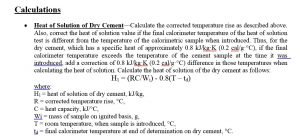# Calculation of Heat of Hydration

Calculations• Heat of Solution of Dry Cement—Calculate the corrected temperature rise as described above. Also, correct the heat of solution value if the final calorimeter temperature of the heat of solution test is different from the temperature of the calorimetric sample when introduced. Thus, for the dry cement, which has a specific heat of approximately 0.8 kJ/kg·K (0.2 cal/g·°C), if the final calorimeter temperature exceeds the temperature of the cement sample at the time it was introduced, add a correction of 0.8 kJ/kg·K (0.2 cal/g·°C) difference in those temperatures when calculating the heat of solution. Calculate the heat of solution of the dry cement as follows:

H1 = (RC/Wi) – 0.8(T – td)

where:
H1 = heat of solution of dry cement, kJ/kg,
R = corrected temperature rise, °C,
C = heat capacity, kJ/°C,
Wi = mass of sample on ignited basis, g,
T = room temperature, when sample is introduced, °C,
td = final calorimeter temperature at end of determination on dry cement, °C.

• Heat of Solution of Partially Hydrated Sample— Calculate the heat of solution of the partially hydrated sample in the same way as for the dry cement except make additional corrections, as follows:
• Since an increase of 1°C in the temperature at which the heat of solution test occurs causes a decrease of approximately 1.3 kJ/kg (0.3 cal/g) in the heat of solution, if the temperature of the heat of solution test of the partially hydrated sample exceeds the temperature of the dry cement determination, a correction of 1.3 kJ/kg·K (°C) difference in temperature shall be added to the heat of solution value obtained for the partially hydrated sample.
• Also, correct the heat of solution value if the final calorimeter temperature of the solution test is different from the temperature of the calorimetric sample when introduced. Thus, for the partially hydrated sample, which has a specific heat of approximately 1.7 kJ/kg (0.4 cal/g) of ignited cement, if the final calorimeter temperature exceeds the temperature of the sample at the time it was introduced, add a correction of 1.7 kJ/kg·K (°C) difference in those temperatures when calculating the heat of solution.
• Calculate the heat of solution of the partially hydrated sample as follows:

H2 = (RC/Wi) – 1.7(T – th) – 1.3(td – th)

where:

H2 = heat of solution of partially hydrated sample, kJ/kg,R, C, Wi,
T = the same definition as above except that they relate to the partially hydrated sample,
td = the same numerical value as in 9.1, and
th = final calorimeter temperature at end of determination on partially hydrated sample, °C.

Heat of Hydration—A final calorimeter temperature of 25°C shall be considered as the basis to which the heat of hydration shall be referred, and the effects of variation in that temperature should be kept in mind when considering test results. An increase in the final temperature raises the heat of hydration approximately 0.4 kJ/kg·K (0.1 cal/g·°C) of ignited cement. For example, if the final temperature is 27°C, 0.8 kJ/kg (0.2 cal/g) should be subtracted from the observed heat of hydration in order to refer the results to 25°C. In borderline cases, proper correction should be made for the effects of final calorimeter temperature. Calculate the heat of hydration of the cement to the nearest kilo joule, as follows:

H = H1 – H2 – 0.4(th – 25.0)

where:
H = heat of hydration of ignited cement, kJ/kg,
H1 = heat of solution of dry cement.
H2 = heat of solution of partially hydrated sample.
th = the same numerical value as above